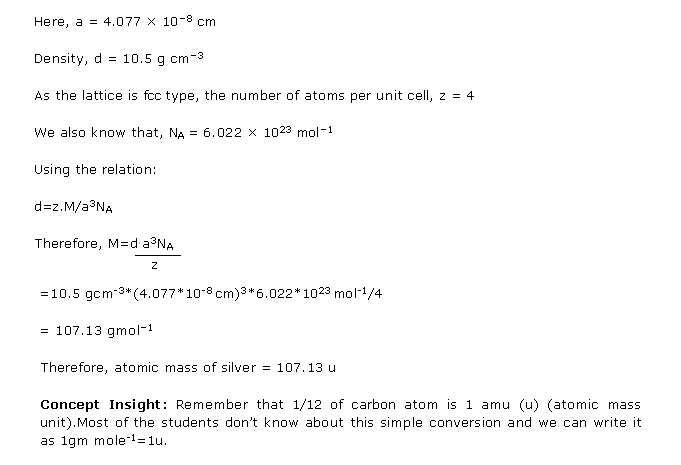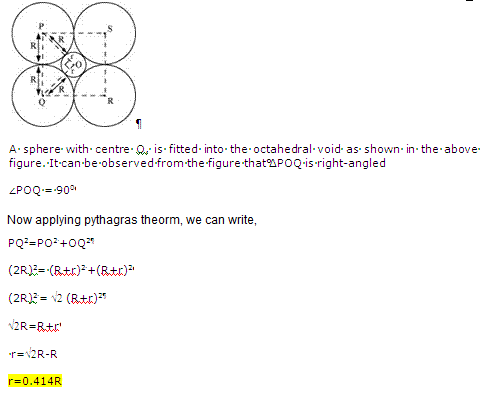# Chapter 1 : The Solid State - Ncert Solutions for Class 12-science Chemistry CBSE

Your Class 12 Science syllabus for Chemistry is designed to make you competent for pursuing higher education in basic sciences, medicine, engineering, etc.  You’ll learn about chemistry concepts such as biomolecules, redox reactions, s-Block elements, thermodynamics, and more. On TopperLearning, you’ll get access to several study resources for learning these concepts well.

Browse through our e-learning resources like sample papers, video lessons, previous year papers, etc. Our video lessons are presented by Chemistry experts to help you enjoy learning Chemistry topics such as properties of colloids, coordination compounds, aldehydes and ketones, DNA, polymers, etc.

Practise likely Chemistry exam questions by using our CBSE Class 12 Science sample papers with solutions and previous paper with solutions. Use our NCERT Chemistry Class 12 solutions for chapter-wise revision. Our MIQs are also beneficial for quick revision of important Chemistry concepts. Study Chemistry concepts and practise our expert solutions for a stress-free Chemistry board exams and score more marks.

CBSE Class 12 Chemistry introduces you to a number of laws, reactions, elements, and other concepts. When you sit to study, you may get confused with the various concepts. The best way to revise Chemistry would be to practise the solutions for Chemistry questions that’s prepared by experts.

On the TopperLearning portal, you’ll find expert solutions to NCERT textbook questions, sample paper questions and questions from previous year CBSE Class 12 Chemistry exam papers. Our Chemistry experts have presented the solutions in a step-wise format to help you understand the solutions. Also, concept notes are added for certain solutions to give you more details about the concept used for answering a question.

NCERT Chemistry Class 12 solutions are important for you to practise different types of questions from your Chemistry textbook. Whether it is a short answer question or a long answer question, you’ll be ready to answer questions accurately in your exam with sufficient practise of the Chemistry solutions.

Page / Exercise

## Chapter 1 - The Solid State Exercise 4

Solution 1Solution 2Solution 3Solution 4Solution 5## Chapter 1 - The Solid State Exercise 6

Solution 1Solution 2Solution 3Solution 4## Chapter 1 - The Solid State Exercise 12

Solution 1Solution 2Solution 3Solution 4## Chapter 1 - The Solid State Exercise 21

Solution 1Solution 2## Chapter 1 - The Solid State Exercise 22

Solution 1Solution 2Solution 3## Chapter 1 - The Solid State Exercise 29

Solution 1Solution 2Solution 3Solution 4Solution 5Solution 6## Chapter 1 - The Solid State Exercise 30

Solution 1Solution 2Solution 3Solution 4Solution 5Solution 6## Chapter 1 - The Solid State Exercise 31

Solution 1Solution 2Solution 3Solution 4Solution 5Solution 6Solution 7Solution 8Solution 9Solution 10Solution 11Solution 12Solution 13Solution 14## Chapter 1 - The Solid State Exercise 32

Solution 1Solution 2Solution 3Solution 4Solution 5Solution 6